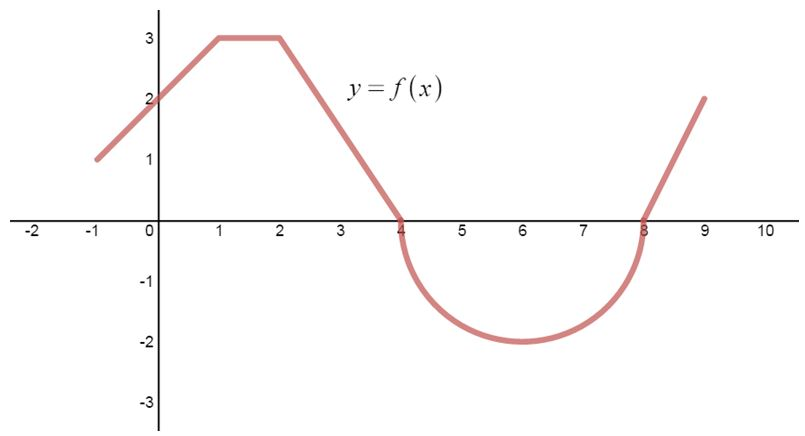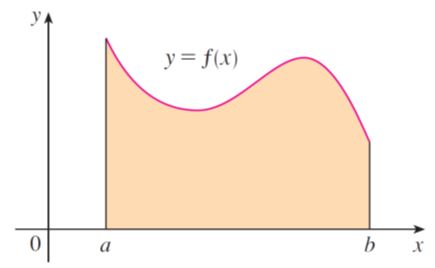# Definite integral

0/10
##### Examples
###### Lessons
1. Use the graph of $f$ to evaluate each integral by interpreting it in terms of areas.1. $\int_{-1}^{1} f(x)dx$
2. $\int_{1}^{2} f(x)dx$
3. $\int_{2}^{4} f(x)dx$
4. $\int_{4}^{8} f(x)dx$
5. $\int_{8}^{9} f(x)dx$
6. $\int_{-1}^{9} f(x)dx$
2. Evaluate the integral by interpreting it in terms of areas.
1. $\int_{0}^{2}(2-2x)dx$
2. $\int_{0}^{2}\sqrt{4-x^2}dx$
3. $\int_{-2}^{1}|x|dx$
3. if $\int_{3}^{10}f(x)dx=15$ and $\int_{3}^{8}f(x)dx=9$, find $\int_{8}^{10}f(x)dx$
###### Free to Join!
StudyPug is a learning help platform covering math and science from grade 4 all the way to second year university. Our video tutorials, unlimited practice problems, and step-by-step explanations provide you or your child with all the help you need to master concepts. On top of that, it's fun - with achievements, customizable avatars, and awards to keep you motivated.
• #### Easily See Your ProgressWe track the progress you've made on a topic so you know what you've done. From the course view you can easily see what topics have what and the progress you've made on them. Fill the rings to completely master that section or mouse over the icon to see more details.
• #### Make Use of Our Learning Aids###### Practice Accuracy

See how well your practice sessions are going over time.

Stay on track with our daily recommendations.

• #### Earn Achievements as You LearnMake the most of your time as you use StudyPug to help you achieve your goals. Earn fun little badges the more you watch, practice, and use our service.
• #### Create and Customize Your AvatarPlay with our fun little avatar builder to create and customize your own avatar on StudyPug. Choose your face, eye colour, hair colour and style, and background. Unlock more options the more you use StudyPug.
###### Topic Notes
In this section, we will evaluate definite integrals by calculating the area under the curve. We see that the region of integration depends on the lower limit and upper limit of the integral. These areas will be fairly easy to calculate since most of the areas under the curve involve shapes that are familiar to us. We will also notice that curves under the x-axis gives us negative area. Next, we will take a look at questions which involves sketching the curve ourselves, and then determining the area. Lastly, we will take a look at a unique question which involves finding the area of a specific region when given information about two definite integrals.$\int_{a}^{b} f(x) dx =$ area under the curve from $a$ to $b$